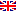Scheda programma d'esame
STATISTICAL METHODS FOR DATA SCIENCE
SALVATORE RUGGIERI
Codice500PP
CFU6
PeriodoSecondo semestre
LinguaInglese

ModuliSettoreTipoOreDocente/i
STATISTICAL METHODS FOR DATA SCIENCESECS-S/01LEZIONI48
 SALVATORE RUGGIERI unimapEsporta in pdf
Obiettivi di apprendimento
Learning outcomes
Conoscenze

The student who completes successfully the course will have a solid knowledge on the main concepts and tools of statistical analysis, including the definition of a statistical model, the inference of its parameters with confidence intervals, the use of hypothesis testing. with specific applications to problems and models useful in data science. Finally the student will be able to use the language R for performing statistical analyses.

Knowledge

The student who completes successfully the course will have a solid knowledge on the main concepts and tools of statistical analysis, including the definition of a statistical model, the inference of its parameters with confidence intervals, the use of hypothesis testing. with specific applications to problems and models useful in data science. Finally the student will be able to use the language R for performing statistical analyses.

Modalità di verifica delle conoscenze

The student will be assessed on his/her demonstrated ability to discuss the main course contents using the appropriate terminology, and to apply the main statistical methods in different contexts.

Assessment criteria of knowledge

The student will be assessed on his/her demonstrated ability to discuss the main course contents using the appropriate terminology, and to apply the main statistical methods in different contexts.

Capacità

The student will be able to understand the main concept of statistical analysis and to choose and apply the appropriate tool to the case under study. The student will also be able to use the language R for performing statistical analyses.

Skills

The student will be able to understand the main concept of statistical analysis and to choose and apply the appropriate tool to the case under study. The student will also be able to use the language R for performing statistical analyses.

Modalità di verifica delle capacità

Attending students will do a group project on the statistical analysis of a large dataset, for which a series of questions will be proposed. The project will assess skills in the choice and use of existing statistical models.

Assessment criteria of skills

Attending students will do a group project on the statistical analysis of a large dataset, for which a series of questions will be proposed. The project will assess skills in the choice and use of existing statistical models.

Comportamenti

Students will be able to evaluate bias in statistical models, particularly in the case of models affecting socially sensitive decision making.

Behaviors

Students will be able to evaluate bias in statistical models, particularly in the case of models affecting socially sensitive decision making.

Modalità di verifica dei comportamenti

Group project and oral exams will include questions about bias in statistical models.

Assessment criteria of behaviors

Group project and oral exams will include questions about bias in statistical models.

Prerequisites

Basic knowledge of calculus. Basic knowledge of probability might be useful even if not indispensable.

Indicazioni metodologiche

Delivery: face to face

Learning activities:

• attending lectures
• participation in discussions
• individual study
• group project

Teaching methods:

• Lectures
• Lab sessions

Teaching methods

Delivery: face to face

Learning activities:

• attending lectures
• participation in discussions
• individual study
• group project

Teaching methods:

• Lectures
• Lab sessions

Programma (contenuti dell'insegnamento)

The program covers the basic methodologies, techniques and tools of statistical analysis. This includes basic knowledge of probability theory, random variables, convergence theorems, statistical models, estimation theory, and hypothesis testing. Other topics covered include bootstrap, expectation-maximization, and applications to data science problems. Finally the program covers the use of the language R for statistical analysis.

Syllabus

The program covers the basic methodologies, techniques and tools of statistical analysis. This includes basic knowledge of probability theory, random variables, convergence theorems, statistical models, estimation theory, and hypothesis testing. Other topics covered include bootstrap, expectation-maximization, and applications to data science problems. Finally the program covers the use of the language R for statistical analysis.

Bibliografia e materiale didattico
•  F.M. Dekking C. Kraaikamp, H.P. Lopuha, L.E. Meester. A Modern Introduction to Probability and Statistics. Springer, 2005.
• P. Dalgaard. Introductory Statistics with R. 2nd edition, Springer, 2008.
Bibliography
•  F.M. Dekking C. Kraaikamp, H.P. Lopuha, L.E. Meester. A Modern Introduction to Probability and Statistics. Springer, 2005.
• P. Dalgaard. Introductory Statistics with R. 2nd edition, Springer, 2008.

Indicazioni per non frequentanti

Non-attending students cannot do the project. All the rest remains unchanged.

Non-attending students info

Non-attending students cannot do the project. All the rest remains unchanged.

Modalità d'esame

The exam consists of a written part and an oral part. The written part includes open questions and exercises. Each exercise is assigned a grade. Students are admitted to the oral part if the sum of grade is at least 18/30. The oral part consists of open questions on the topics of the course. Attending students may replace the written part with a project to be done in groups throughout the course.

Assessment methods

The exam consists of a written part and an oral part. The written part includes open questions and exercises. Each exercise is assigned a grade. Students are admitted to the oral part if the sum of grade is at least 18/30. The oral part consists of open questions on the topics of the course. Attending students may replace the written part with a project to be done in groups throughout the course.

Ultimo aggiornamento 30/07/2018 11:03